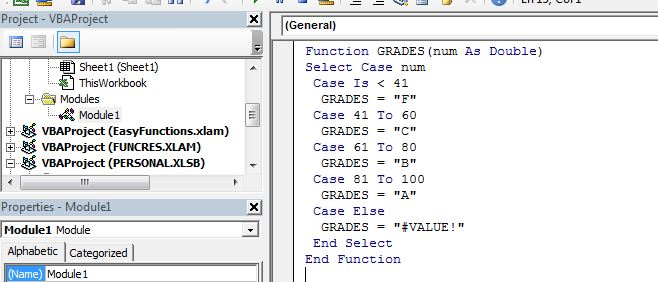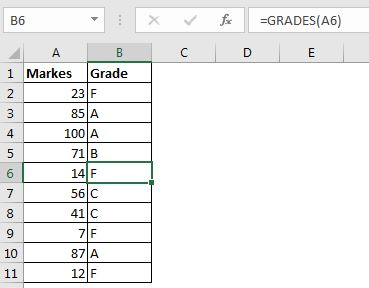# VBA Select Case: An Alternative of Multiple If Else If Statements

When you have many conditions to check or you want to do some operation on the basis of the choice of the user, then you may use If Else If statements. But they are troublesome when it comes to multiple conditions. In that case, the best solution in Excel VBA is the Select Case method.

What is a Select Case statement in VBA?

The Select Case statements in VBA are useful when you have multiple conditions to check. They are like switch statements in other programming languages. It checks the given variable and executes the matched case.

Syntax of Select Case in Excel VBA

```Select Case variable
case Condition1
result1
case Condition2
Condition2
....
case ConditionN
resultN
[case else
resultElse]
```

Select Case: Here Select Case is a keyword to start the case check.

Variable: Variable is any variable that you want to check.

Case: from the next line, we start checking the conditions with variables.

You can have as many conditions as you want. We can check any condition with the keyword case. The condition can be a logical statement or a straight value of the Selected Variable. It will always result in true-false value. These all are valid statements:

``` Select Case region
'using a straight value
Case "Central"
Range("D1").Value = region
'using a is statement
Case Is = "Central"
Range("D1").Value = region
End Select
```

With is you can put arithmetic logical operators to check if the Case is equal to(=), greater than(>), less than(<), etc.

You can use the comma (",") to do one operation on multiple conditions (like or operator).

```Case Is = "West", "North", "South"
Range("D1").Value = region
```

You can also use To operator to check among large ranges.

```Case 1 to 40
Case 41 to 60
Case 61 to 80
Case 81 to 100
```

The Case Else statement is optional. You can use it to do something by default if none of the case match. Like showing a message that the choice is invalid or something.

```Case Else
MsgBox "Invalid Option"

```

Now that we know about the basics of the Select Case, let's have an example.

## Example: Create A Grading Function Using VBA Select Case

We need to create a function that checks the supplied value and returns the grades according to below rules:

• If between 41 and 60, Grade="C"
• If between 61 and 80, Grade="B"
• If between 81 and 100, Grade="A"
• else #VALUE! error.

We will use the Select Case as a Switch statement of VBA here. The code for the custom function will be:

```Function GRADES(num As Double)
Select Case num
Case Is < 41
Case 41 To 60
Case 61 To 80
Case 81 To 100
Case Else
End Select
End Function

```Now if you use this function on the sheet, it will return the grades easily.So yeah guys, this is how the Select Case (switch) statement is used in Excel VBA to check multiple conditions. Instead of multiple If Else If statement we use the Select Case statement to switch results. If you have any doubts regarding this article or any other Excel/VBA related articles, ask in the comments section below.Related Articles:

Using Loop in VBA in Microsoft Excel | The loops in VBA enable us to do a similar task over and over without repetition of code. There are 3 types of loops in Excel VBA.

7 Examples of For Loops in Microsoft Excel VBA | The 7 examples of for loop can make your automation life easy. Let's start with simple for loop.

Popular Articles:

50 Excel Shortcuts to Increase Your Productivity | Get faster at your task. These 50 shortcuts will make you work even faster on Excel.

The VLOOKUP Function in Excel | This is one of the most used and popular functions of excel that is used to lookup value from different ranges and sheets.

COUNTIF in Excel 2016 | Count values with conditions using this amazing function. You don't need filter your data to count specific value. Countif function is essential to prepare your dashboard.

How to Use SUMIF Function in Excel | This is another dashboard essential function. This helps you sum up values on specific conditions.

## Users are saying about us...

1.Christopher Mackay

The grading example can also be written
Select Case num
Case Is < 41: GRADES = "F"
Case is < 61: GRADES = "C"
Case is < 81: GRADES = "B"
Case is <= 100: GRADES = "A"
•